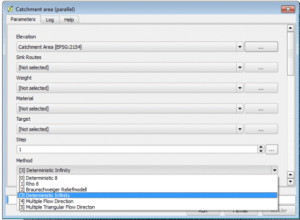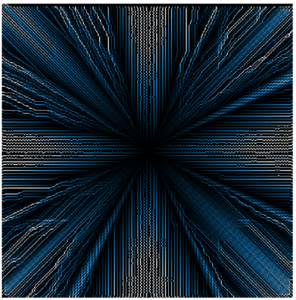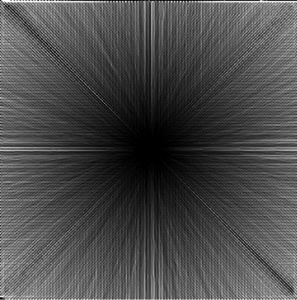### Hydrology with a GIS, for the Dummies (that we are): calculation of the flow (3)

In the two previous articles ( Hydrology with a GIS, for Dummies (that we are): calculation of the flow (1)   and   Hydrology with a GIS, for the nulls (that we are): calculation of the flow (2 ) we have approached three methods to calculate the flow of flow: two one-dimensional methods (D8 and Rho8) and a two-dimensional method (MFD).

Now we will discuss a variation of the most widespread method (D8) which we addressed first.

Fourth Method: Infinite Deterministic (D∞)

The difference between this method and the D8 method is that the flux is distributed in the two lowest adjacent cells and not just in the lowest cell. It is a two-dimensional method.

In QGis you will use, in the processing window- > SAGA -> Terrain analysis-Hydrology -> Catchment area (parallel)The result of the calculation of the flow with this method, on the DEM involves a semi-sphere, as it follows:If we compare this with the result of the D8 method:We can see that, although the problem of the overrepresentation in the 8 directions (0 , 45,90 , …) is always very marked, the differences are dimmed.

The ratio of cells with less surface area to those with larger surface area is from 1 to 700 in the case of the D8 method, and only from 1 to 22 in the case of the infinite deterministic method.

Fifth method: kinematic routing algorithm (KRA)

In this section we can mention the KRA method: the kinematic routing algorithm. This flow  algorithm is one-dimensional. The flow behaves like a ball rolling on the DTM, without restricting its position in the centre of the cells. This result is intermediate compared to the two previous methods. The ratio among the cells with less surface area to those with larger surface area is from 1 to 45.We will discuss briefly the remaining methods since they do not provide much more information.

Sixth method: Braunschweiger Digital Reliefmodell

This is a multidimensional method. It is similar to the MFD method.
It is available in QGis in the Treatment Window- > SAGA -> Terrain analysis-Hydrology -> Catchment area ( parallel )
The result of the calculation is as follows:This result has to be compared with the result of the MFD method:The result appears much better than those produced by the one-dimensional methods, but compared to the MFD method we can see a certain radial bias.

Seventh method: DEMON (Digital Elevation Model Network)

It is a two-dimensional method, but rather complicated. It is based on the creation of an upstream and downstream watershed matrix for each pixel. Impossible to explain it here. If you are interested, it is better to go to the source ( Costa-Cabral, MC; Burges , S. J. Digital elevation model networks (DEMON): A model of flow over hills for computation of contributing and dispersal areas, Wat Resour . Res 30: 1681-92 (1994 )
Just remember that it takes a long time to calculate the result. In the case of our example, which is very simple, it takes about 8 minutes to calculate the result. So with real MNTs …
In QGis it is available in QGis in Process Window- > SAGA -> Terrain analysis-Hydrology -> Catchment area (flow tracing )
Here is the result:This result is very close to the infinite deterministic method (see the beginning of this article), which is normal since both are two-dimensional methods.

We have gone through the main methods for calculating the flow. It remains to discuss in more detail the problems posed by the main methods. This is what we will discuss in the next and last article on this subject